# Regular polyhedra

Platonic solids

Convex polyhedra such that all faces are congruent regular polygons and such that all polyhedral angles at the vertices are regular and equal (Fig.1a–Fig.1e).Figure: r080790aFigure: r080790b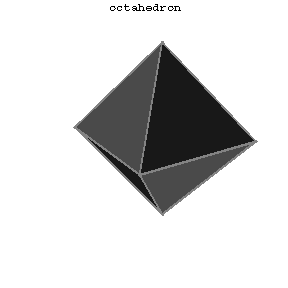Figure: r080790c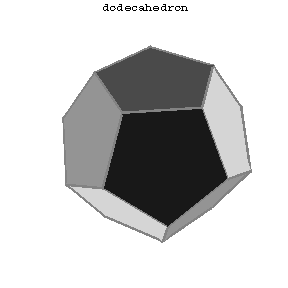Figure: r080790d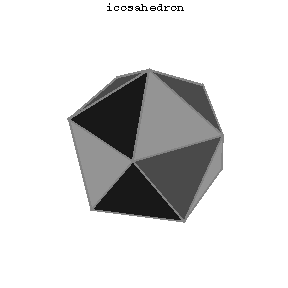Figure: r080790e

In the Euclidean space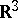there are five regular polyhedra, the data of which are given in Table 1, where the Schläfli symbol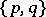(cf. Polyhedron group) denotes the regular polyhedron with-gonal faces and-hedral angles'
<tbody> </tbody>
 Figure Schläfli symbol # vertices # edges # faces Tetrahedron 1a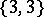4 6 4 Cube (hexahedron) 1b8 12 6 Octahedron 1c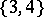6 12 8 Dodecahedron 1d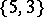20 30 12 Icosahedron 1e12 30 20

Dual polyhedra, or reciprocal polyhedra,and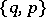are, by definition, those which transform into each other upon reciprocation with respect to any concentric sphere. The tetrahedron is dual to itself, the hexahedron to the octahedron and the dodecahedron to the icosahedron.

Polyhedra in spaces of more than three dimensions are called polytopes.

Inthere are six regular polytopes, the data of which are given in Table 2.'
<tbody> </tbody>
 Schläfli symbol # vertices # edges #-dim. faces #-dim. faces Simplex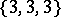5 10 10 5 4-Cube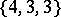16 32 24 8 16-cell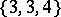8 24 32 16 24-cell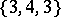24 96 96 24 120-cell600 1200 720 120 600-cell120 720 1200 600

In,, there are three regular polytopes: the analogues of the tetrahedron, the cube and the octahedron; their Schläfli symbols are:,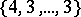and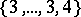.

If one permits self-intersection, then there aremore regular polyhedra, namely the Kepler–Poinsot solids or regular star polyhedra. In these polyhedra, either the faces intersect each other or the faces are self-intersecting polygons (Fig.2a–Fig.2d). The data of these solids are listed in Table 3.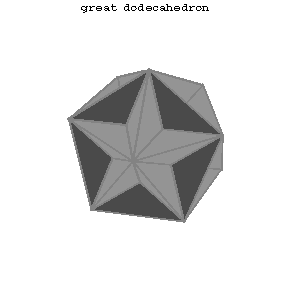Figure: r080790f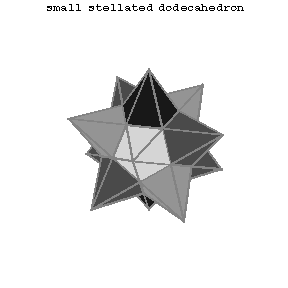Figure: r080790gFigure: r080790hFigure: r080790i

'
<tbody> </tbody>
 Figure # vertices # edges # faces Great dodecahedron 2a 12 30 12 Small stellated dodecahedron 2b 12 30 12 Great stellated dodecahedron 2c 20 30 12 Great icosahedron 2d 12 30 20